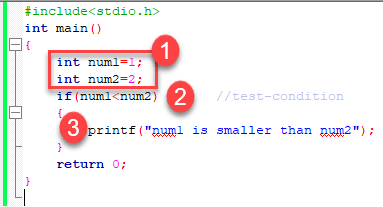if else statement in c

# If Else Statement in C

``` if (condition)
instruction;```

The condition evaluates to either true or false. True is always a non-zero value, and false is a value that contains zero. Instructions can be a single instruction or a code block enclosed by curly braces { }.

Following program illustrates the use of if construct in ‘C’ programming:

```#include<stdio.h>
int main()
{
int num1=1;
int num2=2;
if(num1<num2)		//test-condition
{
printf("num1 is smaller than num2");
}
return 0;
}
```

Output:

```num1 is smaller than num2
```

The above program illustrates the use of if construct to check equality of two numbers.1. In the above program, we have initialized two variables with num1, num2 with value as 1, 2 respectively.
2. Then, we have used if with a test-expression to check which number is the smallest and which number is the largest. We have used a relational expression in if construct. Since the value of num1 is smaller than num2, the condition will evaluate to true.